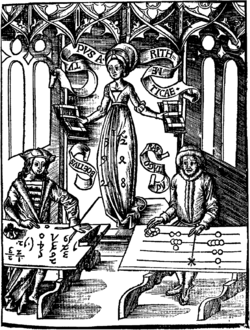# Algorism

﻿
AlgorismCalculating-Table by Gregor Reisch: Margarita Philosophica, 1508

Algorism is the technique of performing basic arithmetic by writing numbers in place value form and applying a set of memorized rules and facts to the digits. One who practices algorism is known as an algorist. This system largely superseded earlier calculation systems that used a different set of symbols for each numerical magnitude and in some cases required a device such as an abacus.

## Etymology

The word "Algorism", comes from the name Al-Khwārizmī (c. 780-850), a Persian mathematician, astronomer, a geographer and a scholar in the House of Wisdom in Baghdad, whose name means "the native of Kharazm", a city that was part of the Greater Iran during his era and now is in modern day Uzbekistan He wrote a treatise in Arabic language in the 9th century, which was translated into Latin in the 12th century under the title Algoritmi de numero Indorum. This title means "Algoritmi on the numbers of the Indians", where "Algoritmi" was the translator's Latinization of Al-Khwarizmi's name. Al-Khwarizmi was the most widely read mathematician in Europe in the late Middle Ages, primarily through his other book, the Algebra. In late medieval Latin, algorismus, the corruption of his name, simply meant the "decimal number system" that is still the meaning of modern English algorism. In 17th century French the word's form, but not its meaning, changed to algorithm, following the model of the word logarithm, this form alluding to the ancient Greek arithmos = number. English adopted the French very soon afterwards, but it wasn't until the late 19th century that "algorithm" took on the meaning that it has in modern English. In English, it was first used about 1230 and then by Chaucer in 1391. Another early use of the word is from 1240, in a manual titled Carmen de Algorismo composed by Alexandre de Villedieu. It begins thus:

"Haec algorismus ars praesens dicitur, in qua Talibus Indorum fruimar bis quinque figuris."

"Algorism is the art by which at present we use those Indian figures, which number two times five."

The word Algorithm also derives from "Algorism", a generalization of the meaning to any set of rules specifying a computational procedure. Occasionally algorism is also used in this generalized meaning, especially in older texts.

## History

Starting with the integer arithmetic developed in India using base 10 notation, Al-Khwārizmī along with other mathematicians in medieval Islam, both Iranian and Arabic, documented new arithmetic methods and made many other contributions to decimal arithmetic (see the articles linked below). These included the concept of the decimal fractions as an extension of the notation, which in turn led to the notion of the decimal point.

Wikimedia Foundation. 2010.

### Look at other dictionaries:

• Algorism — Al go*rism, Algorithm Al go*rithm, n. [OE. algorism, algrim, augrim, OF. algorisme, F. algorithme (cf. Sp. algoritmo, OSp. alguarismo, LL. algorismus), fr. the Ar. al Khow[=a]rezm[=i] of Khow[=a]rezm, the modern Khiwa, surname of Abu Ja far… …   The Collaborative International Dictionary of English

• algorism — [al′gə riz΄əm] n. [ME & OFr algorisme < ML algorismus, after Ar al Khwārazmī, lit., native of Khwārazm (Khiva), mathematician of the 9th c. A.D.] 1. the Arabic system of numerals; decimal system of counting 2. the act or skill of computing… …   English World dictionary

• algorism — noun Computing in, or using, Arabic numerals. The Algorism in Addition of Fractions. There are two accepted forms of work in addition of fractions …   Wiktionary

• algorism — algorismic, adj. /al geuh riz euhm/, n. Math. Now Rare. 1. the Arabic system of arithmetical notation (with the figures 1, 2, 3, etc.). 2. the art of computation with the Arabic figures, one to nine, plus the zero; arithmetic. 3. algorithm. [1200 …   Universalium

• Algorism — System of Arabic numeration, arithmetic. European acquaintance with and adoption of Arabic numerals came from translations of Arab mathematicians, esp. in Moorish Spain. [< Ar. al Khwarizmi = a 9c Arab mathematician; thus also, algorithm] Cf.… …   Dictionary of Medieval Terms and Phrases

• algorism — n. decimal numeral system; numeration with Arabic numerals …   English contemporary dictionary

• algorism — al·go·rism …   English syllables

• algorism — al•go•rism [[t]ˈæl gəˌrɪz əm[/t]] n. math. the Arabic system of arithmetical notation (with the figures 1, 2, 3, etc.) • Etymology: 1200–1250; ME augrim, algrim < MF < ML algorismus < Ar al the +kh(u) wārizmī (surname of a 9th cent.… …   From formal English to slang

• algorism — /ˈælgərɪzəm/ (say alguhrizuhm) noun 1. the Arabic system of arithmetical notation (with the figures 1, 2, 3, etc.). 2. the art of computation with the Arabic figures, one to nine, plus the zero; arithmetic. 3. → algorithm. {Middle English… …   Australian English dictionary

• algorism —   n. art of calculating, especially in Arabic numeration; arithmetic.    ♦ algorismic, a …   Dictionary of difficult words